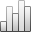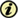Acta Stereologica

0351-580XPlus de Statistiquesdepuis le 05 décembre 2013 :
Visualisation(s): 155 (1 ULiège)
Téléchargement(s): 32 (0 ULiège)

### A relationship between the parameter κ of a von mises distribution and intersection densities measured on two perpendicular test lines

(Volume 3 (1984) — Number 1 - May 1984)
Article#### Abstract

The fraction of the two intersection densities of test lines along and perpendicular to a known prevalent direction is considered as a function of the concentration parameter κ of the bimodal von Mises distribution. This is done similar to Weibel (1980), who derived the corresponding function for the Mariott distribution. A table and the asymptotic behaviour are given. Small deviations of the test line system to the prevalent direction are considered. Finally it is shown, that a three dimensional initial problem, raised by biopedologists can be treated with the appropriate formula and also with the results given in the paper of Mathieu et al. (1983), depending on the assumed model.

#### Pour citer cet article

Konrad Sandau, «A relationship between the parameter κ of a von mises distribution and intersection densities measured on two perpendicular test lines», Acta Stereologica [En ligne], Volume 3 (1984), Number 1 - May 1984, 11-17 URL : https://popups.ulg.ac.be/0351-580x/index.php?id=3908.

#### A propos de : Konrad Sandau

Institut für Angewandte Mathematik und Statistik, Universität Hohenheim, Pf. 700562/110, 7000 Stuttgart 70, FRG